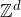# N.Hao & M. Heydenreich: Distances in Scale-free Percolation

Abstract: We study an inhomogeneous version of long-range percolation known as scale-free percolation. Every vertex inis equipped with an i.i.d. weight, and two vertices are linked by an edge with probability depending on the Euclidean distance and the weight of the two vertices involved. This model, introduced by Deijfen, van der Hofstad and Hooghiemstra in 2013, exhibits a rich phase diagram w.r.t. graph distances and percolation properties. In the first part of the talk, we introduce the model, describe some of the recent developments and discuss a far-reaching generalisation. The main focus of the talk is then on graph distances, in particular in a regime where the graph distance between two vertices is (poly-)logarithmic w.r.t. the Euclidean distance. We explain how a hierarchical argument developed by Biskup (2004) can be extended to the inhomogeneous setting.

This talk is based on the preprint https://arxiv.org/abs/2105.05709.

## Video